The dbscan package  includes a fast implementation of Hierarchical DBSCAN (HDBSCAN) and its related algorithm(s) for the R platform. This vignette introduces how to interface with these features. To understand how HDBSCAN works, we refer to an excellent Python Notebook resource that goes over the basic concepts of the algorithm (see the SciKit-learn docs). For the sake of simplicity, consider the same sample dataset from the notebook:

``````library("dbscan")
data("moons")
plot(moons, pch=20)``````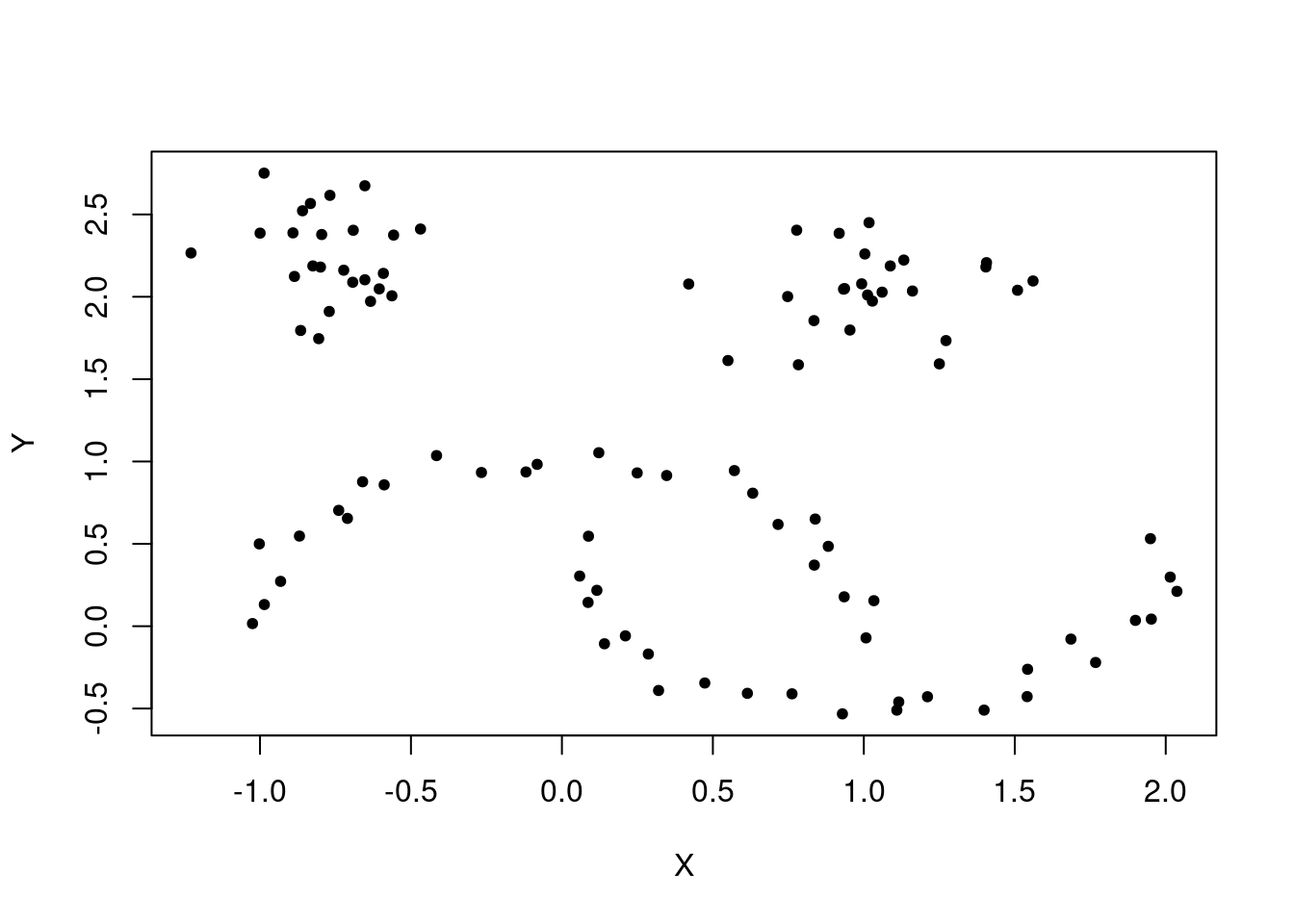To run the HDBSCAN algorithm, simply pass the dataset and the (single) parameter value ‘minPts’ to the hdbscan function.

``````  cl <- hdbscan(moons, minPts = 5)
cl``````
``````## HDBSCAN clustering for 100 objects.
## Parameters: minPts = 5
## The clustering contains 3 cluster(s) and 0 noise points.
##
##  1  2  3
## 25 25 50
##
## Available fields: cluster, minPts, coredist, cluster_scores,
##                   membership_prob, outlier_scores, hc``````

The ‘flat’ results are stored in the ‘cluster’ member. Noise points are given a value of 0, so increment by 1.

`` plot(moons, col=cl\$cluster+1, pch=20)``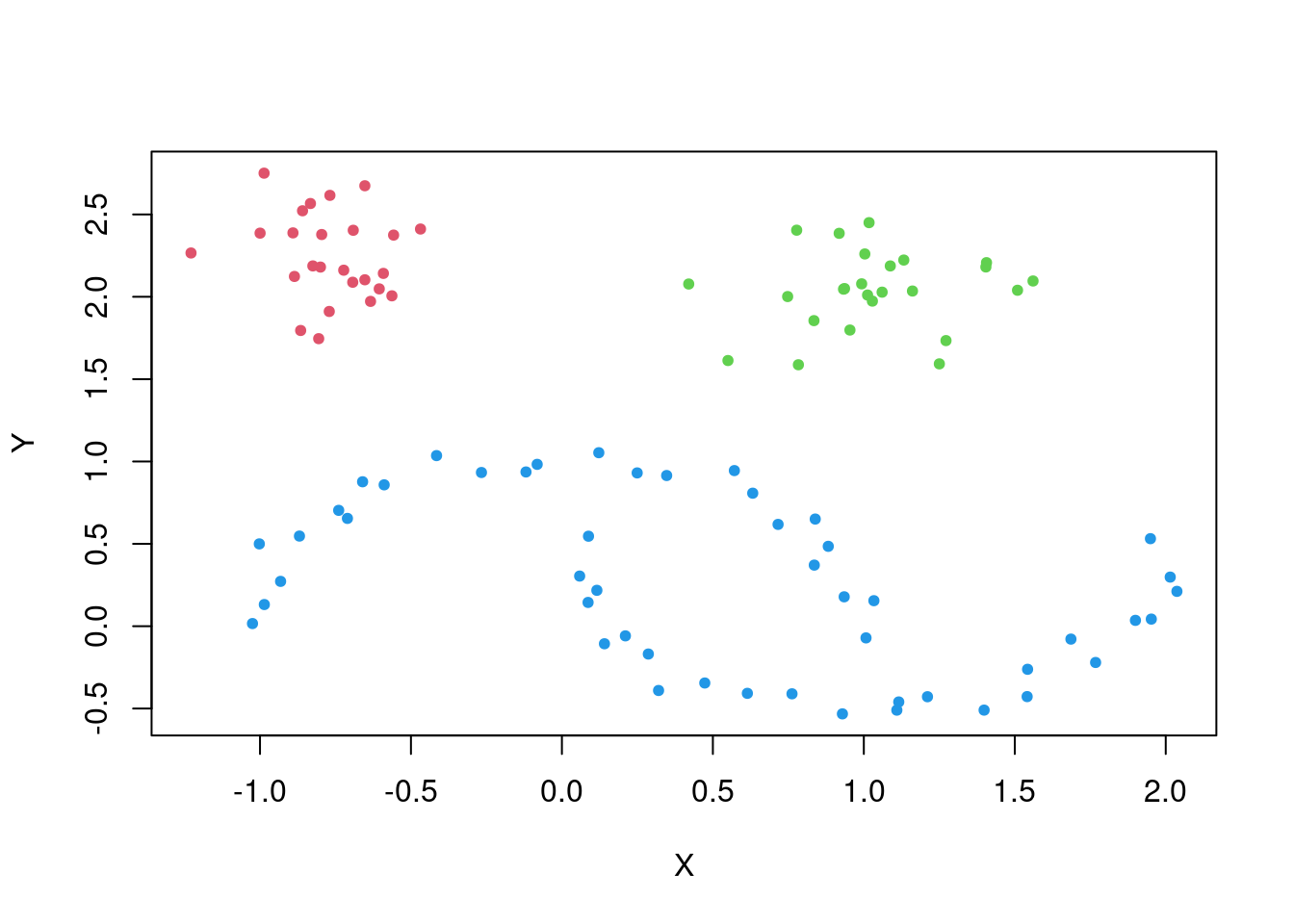The results match intuitive notions of what ‘similar’ clusters may look like when they manifest in arbitrary shapes.

## Hierarchical DBSCAN

The resulting HDBSCAN object contains a hierarchical representation of every possible DBSCAN* clustering. This hierarchical representation is compactly stored in the familiar ‘hc’ member of the resulting HDBSCAN object, in the same format of traditional hierarchical clustering objects formed using the ‘hclust’ method from the stats package.

``cl\$hc``
``````##
## Call:
## hdbscan(x = moons, minPts = 5)
##
## Cluster method   : robust single
## Distance         : mutual reachability
## Number of objects: 100``````

Note that although this object is available for use with any of the methods that work with ‘hclust’ objects, the distance method HDBSCAN uses (mutual reachability distance, see ) is not an available method of the hclust function. This hierarchy, denoted the “HDBSCAN* hierarchy” in , can be visualized using the built-in plotting method from the stats package

``plot(cl\$hc, main="HDBSCAN* Hierarchy")``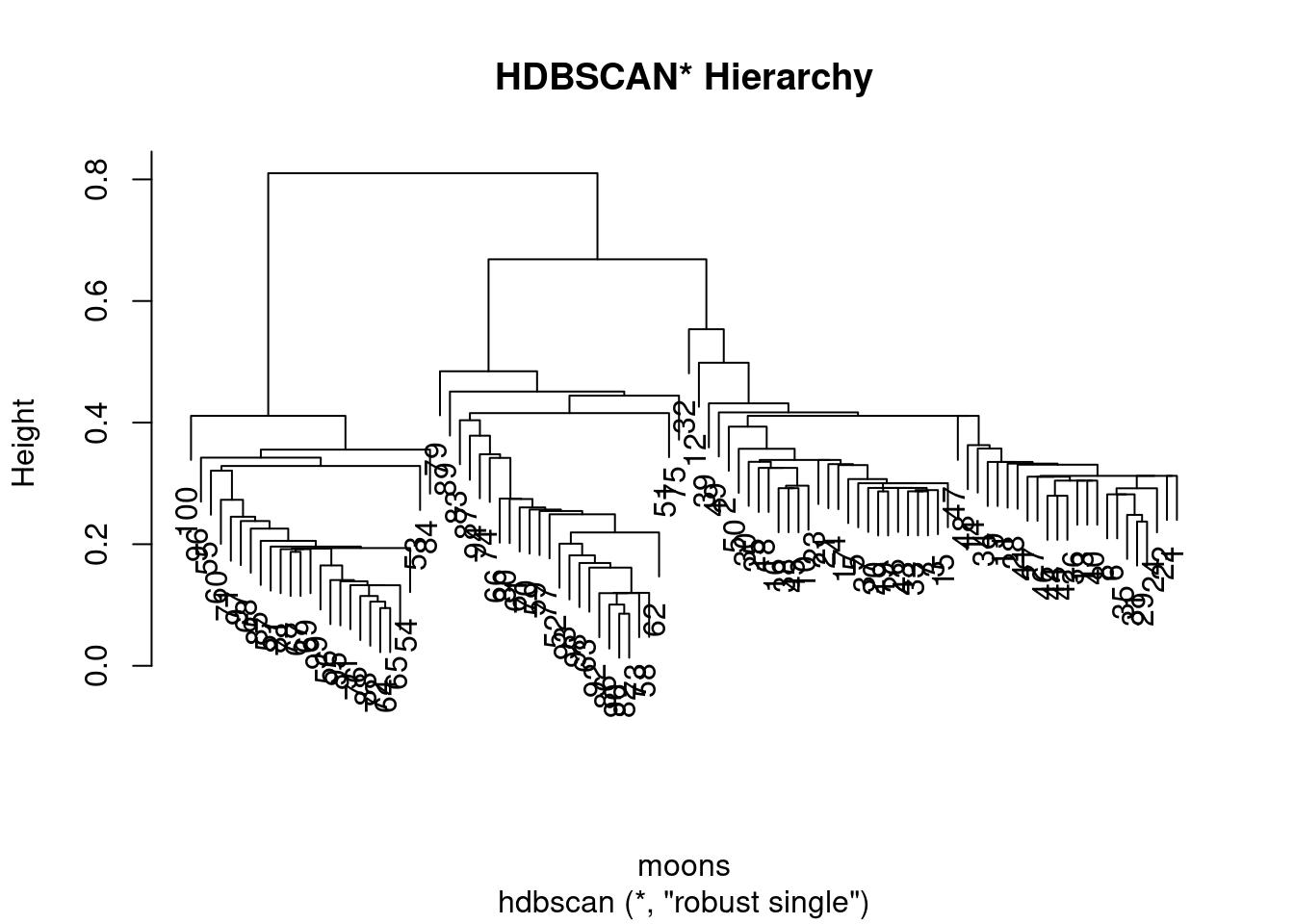## DBSCAN* vs cutting the HDBSCAN* tree

As the name implies, the fascinating thing about the HDBSCAN* hierarchy is that any global ‘cut’ is equivalent to running DBSCAN* (DBSCAN w/o border points) at the tree’s cutting threshold \(eps\) (assuming the same \(minPts\) parameter setting was used). But can this be verified manually? Using a modified function to distinguish noise using core distance as 0 (since the stats cutree method does not assign singletons with 0), the results can be shown to be identical.

``````cl <- hdbscan(moons, minPts = 5)
check <- rep(F, nrow(moons)-1)
core_dist <- kNNdist(moons, k=5-1)

## cutree doesn't distinguish noise as 0, so we make a new method to do it manually
cut_tree <- function(hcl, eps, core_dist){
cuts <- unname(cutree(hcl, h=eps))
cuts[which(core_dist > eps)] <- 0 # Use core distance to distinguish noise
cuts
}

eps_values <- sort(cl\$hc\$height, decreasing = T)+.Machine\$double.eps ## Machine eps for consistency between cuts
for (i in 1:length(eps_values)) {
cut_cl <- cut_tree(cl\$hc, eps_values[i], core_dist)
dbscan_cl <- dbscan(moons, eps = eps_values[i], minPts = 5, borderPoints = F) # DBSCAN* doesn't include border points

## Use run length encoding as an ID-independent way to check ordering
check[i] <- (all.equal(rle(cut_cl)\$lengths, rle(dbscan_cl\$cluster)\$lengths) == "TRUE")
}
print(all(check == T))``````
``##  TRUE``

## Simplified Tree

The HDBSCAN* hierarchy is useful, but for larger datasets it can become overly cumbersome since every data point is represented as a leaf somewhere in the hierarchy. The hdbscan object comes with a powerful visualization tool that plots the ‘simplified’ hierarchy(see  for more details), which shows cluster-wide changes over an infinite number of \(eps\) thresholds. It is the default visualization dispatched by the ‘plot’ method

`` plot(cl)``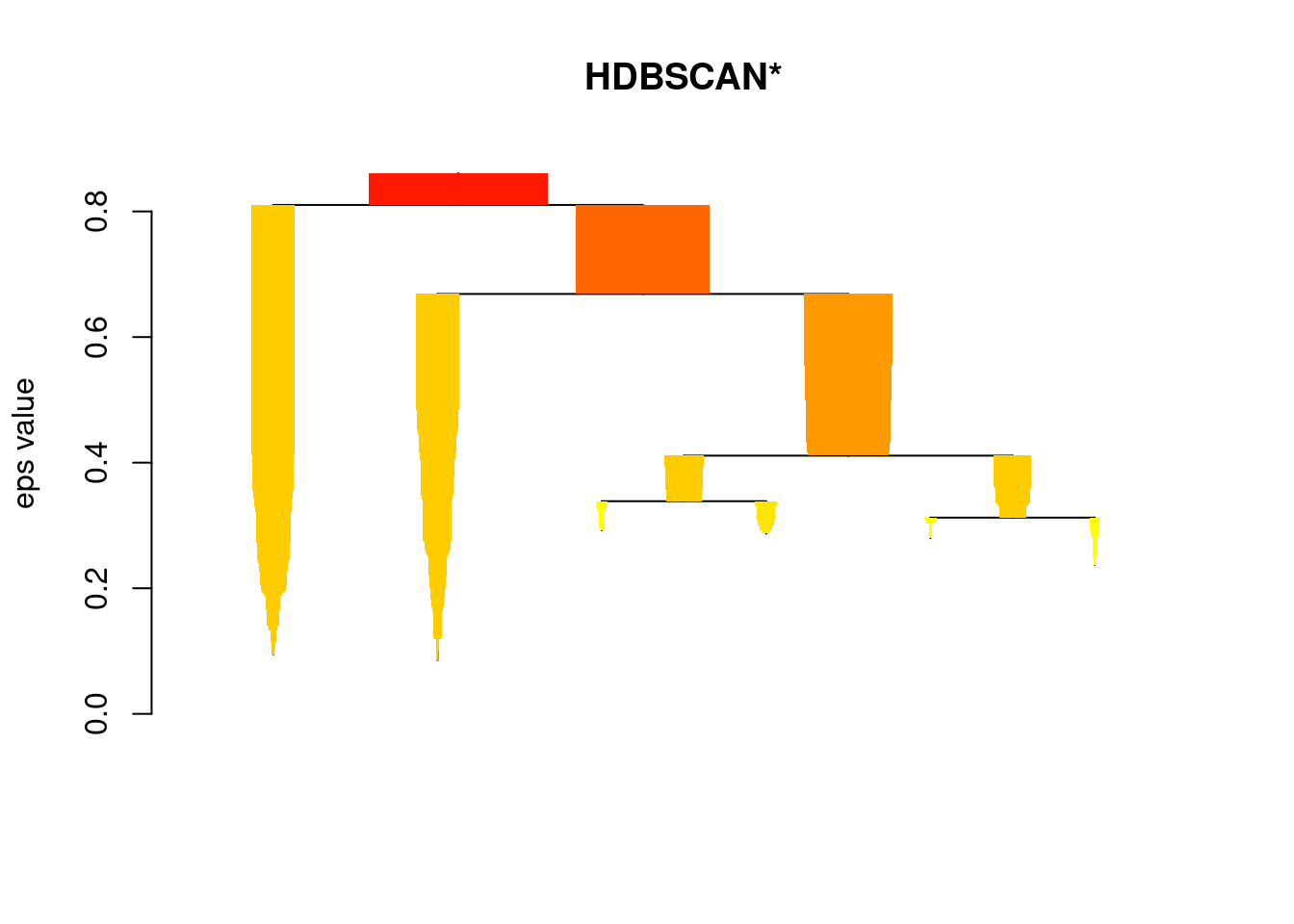You can change up colors

`` plot(cl, gradient = c("yellow", "orange", "red", "blue"))``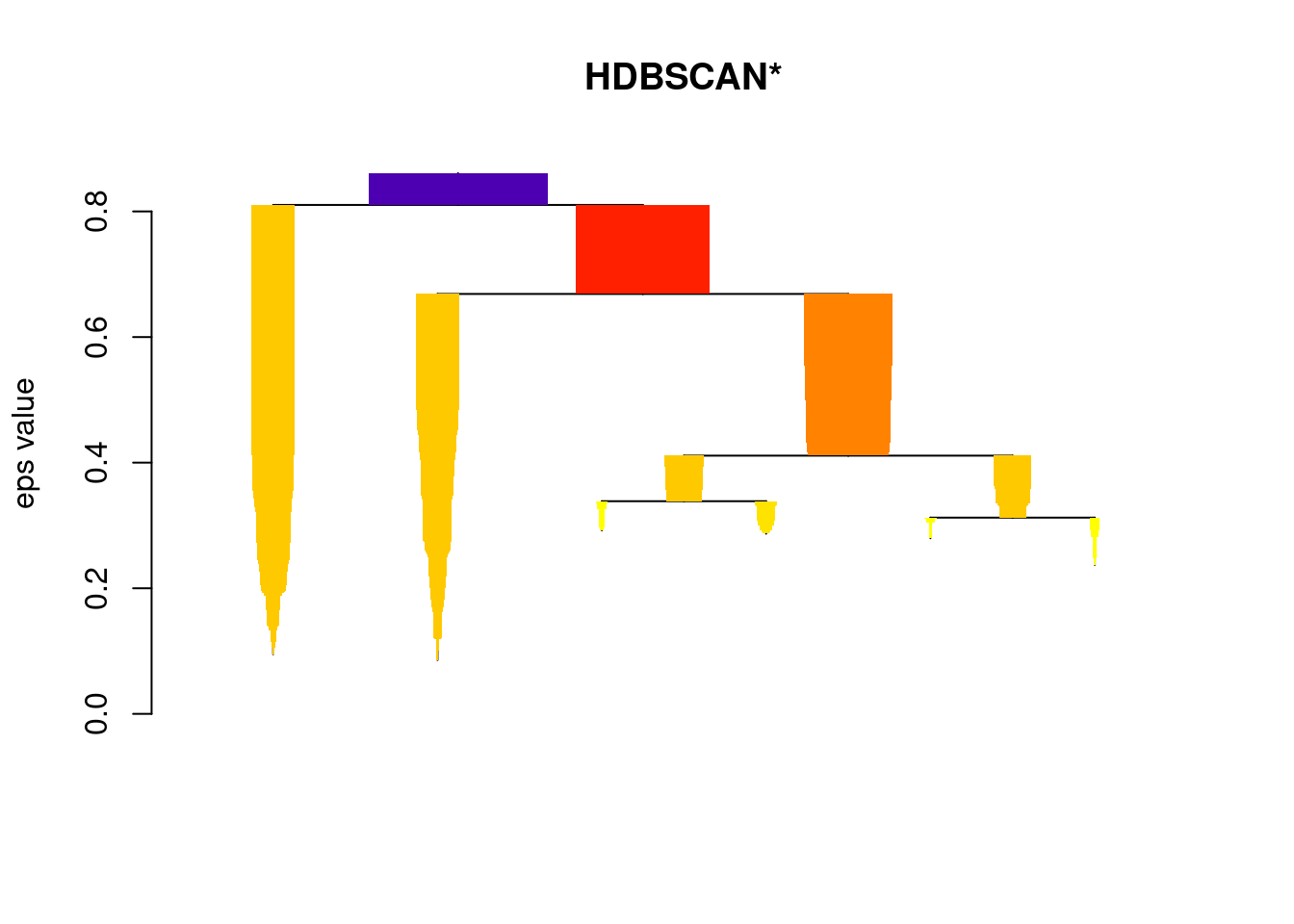… and scale the widths for individual devices appropriately

``plot(cl, gradient = c("purple", "blue", "green", "yellow"), scale=1.5)``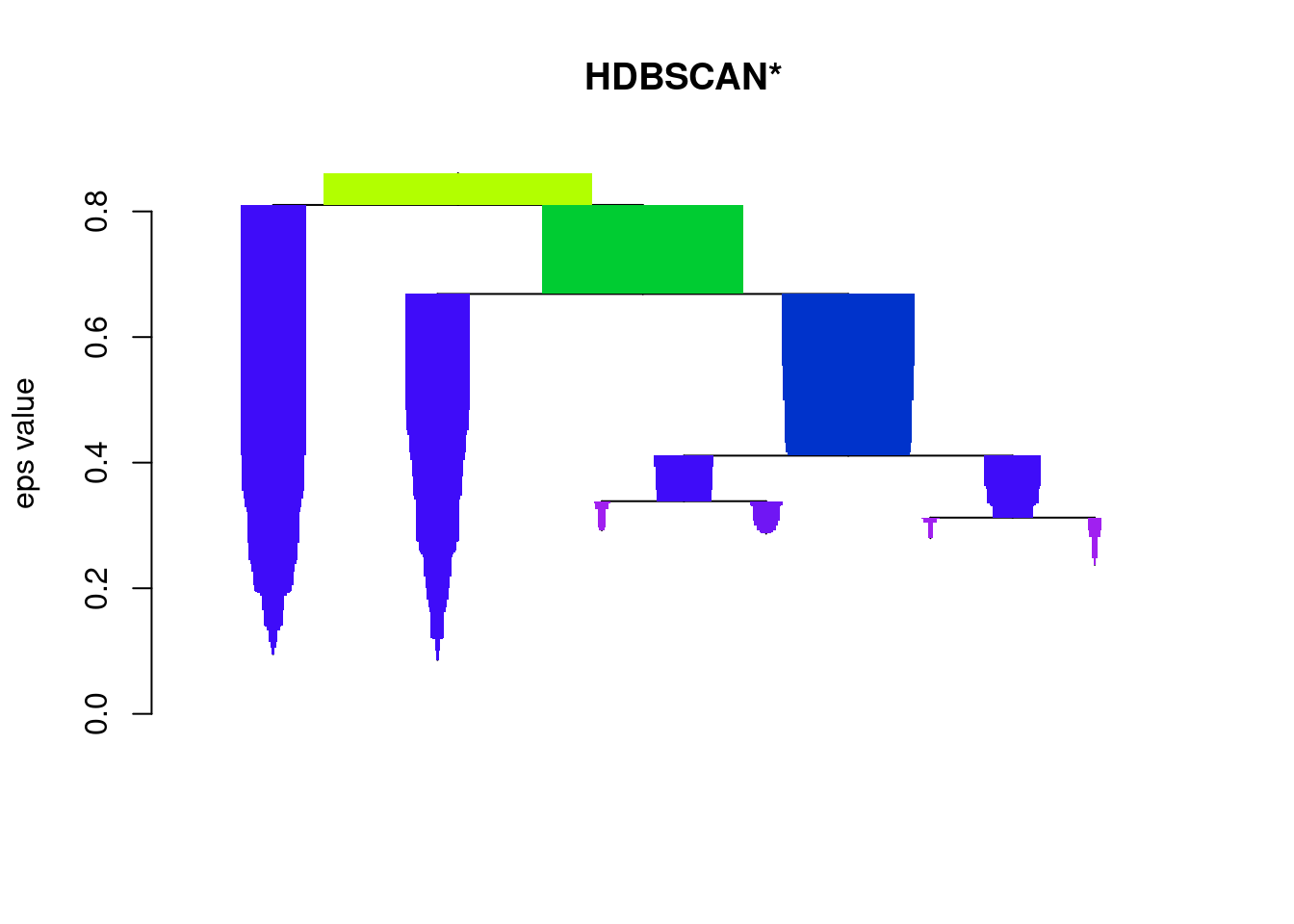… even outline the most ‘stable’ clusters reported in the flat solution

``plot(cl, gradient = c("purple", "blue", "green", "yellow"), show_flat = T)``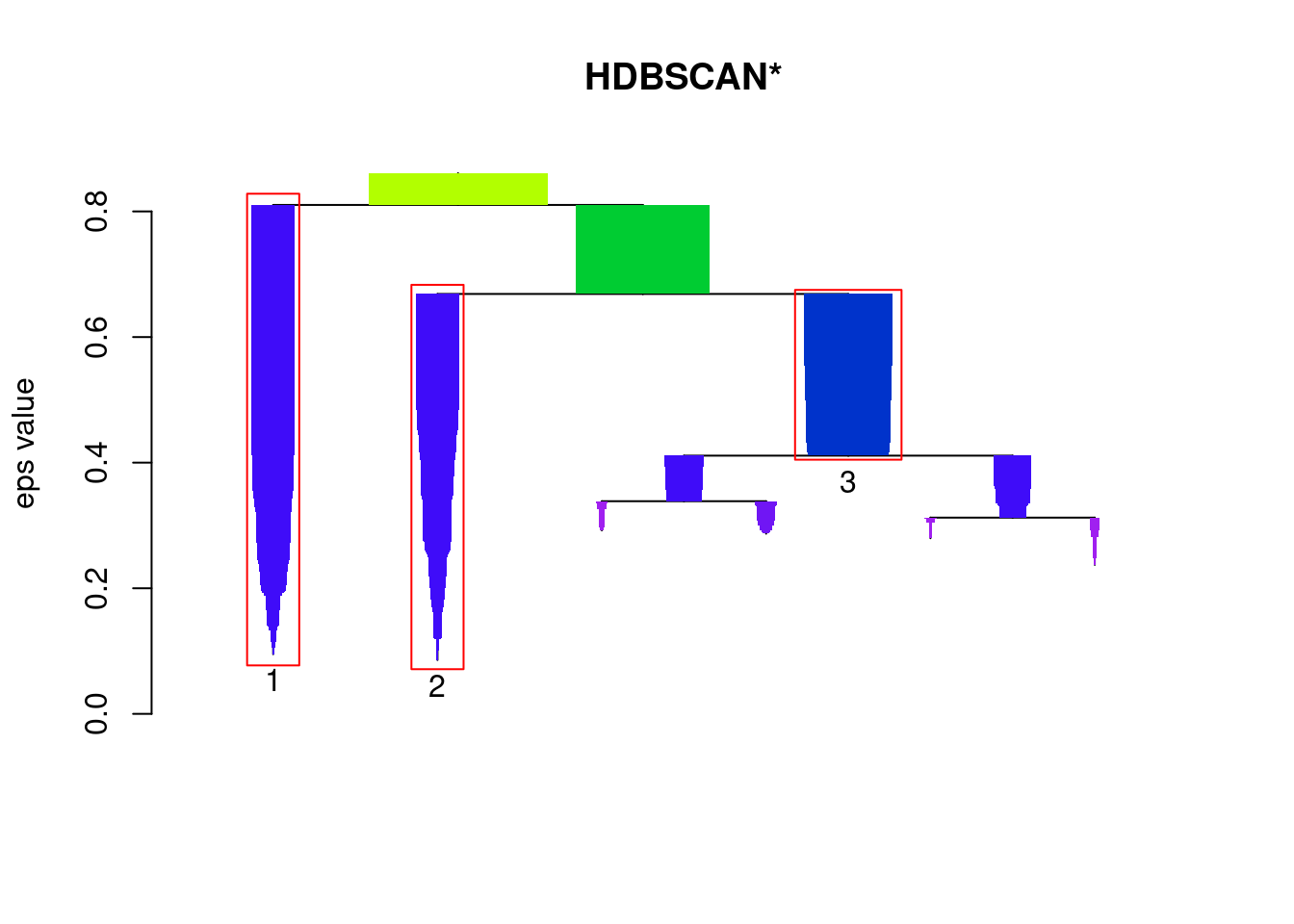## Cluster Stability Scores

Note the stability scores correspond to the labels on the condensed tree, but the cluster assignments in the cluster member element do not correspond to the labels in the condensed tree. Also, note that these scores represent the stability scores before the traversal up the tree that updates the scores based on the children.

``print(cl\$cluster_scores)``
``````##         1         2         3
## 110.70613  90.86559  45.62762``````

The individual point membership ‘probabilities’ are in the probabilities member element

``  head(cl\$membership_prob)``
``##  0.4354753 0.2893287 0.4778663 0.4035933 0.4574012 0.4904582``

These can be used to show the ‘degree of cluster membership’ by, for example, plotting points with transparencies that correspond to their membership degrees.

``````  plot(moons, col=cl\$cluster+1, pch=21)
colors <- mapply(function(col, i) adjustcolor(col, alpha.f = cl\$membership_prob[i]),
palette()[cl\$cluster+1], seq_along(cl\$cluster))
points(moons, col=colors, pch=20)``````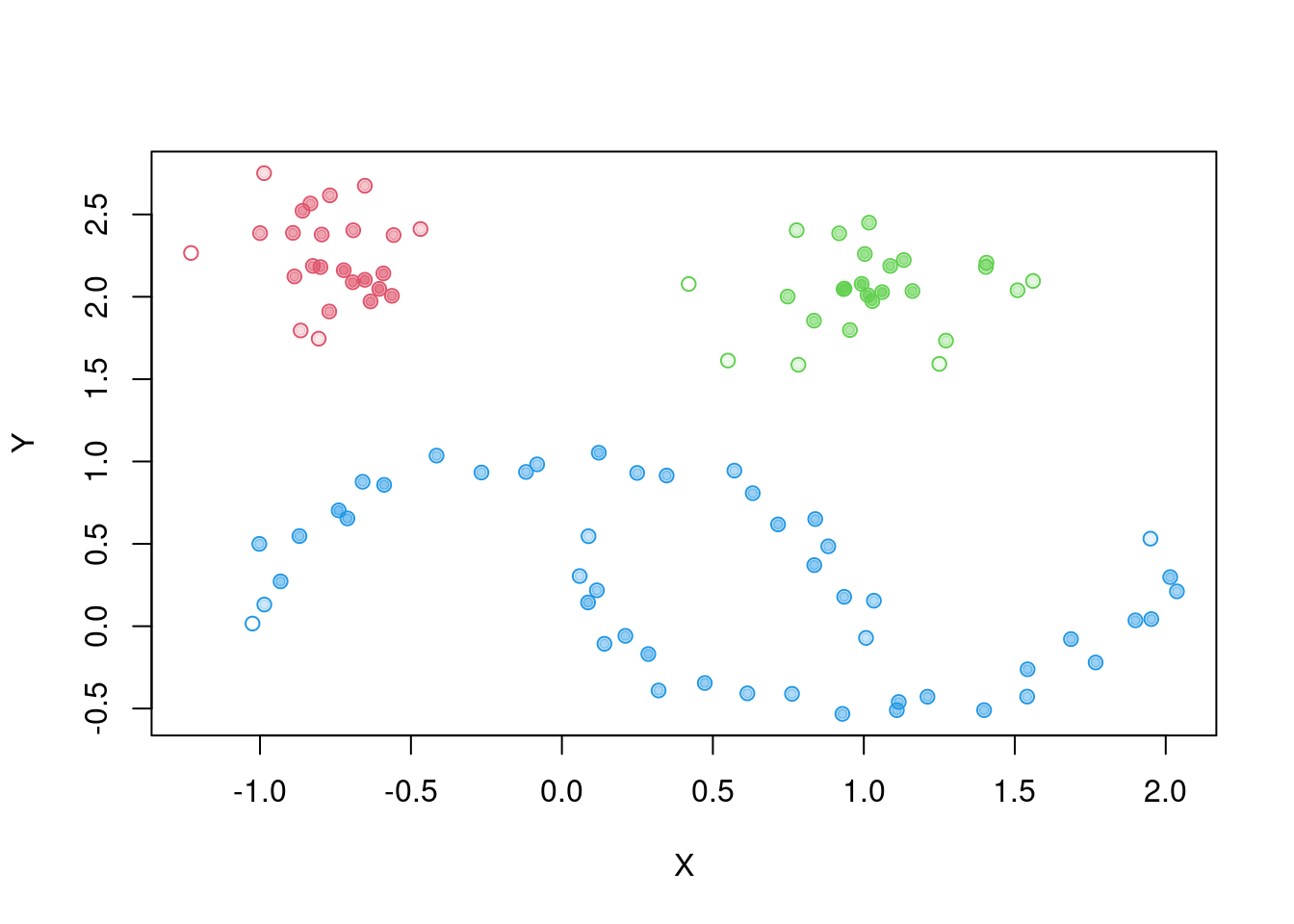## Global-Local Outlier Score from Hierarchies

A recent journal publication on HDBSCAN comes with a new outlier measure that computes an outlier score of each point in the data based on local and global properties of the hierarchy, defined as the Global-Local Outlier Score from Hierarchies (GLOSH). An example of this is shown below, where unlike the membership probabilities, the opacity of point represents the amount of “outlierness” the point represents. Traditionally, outliers are generally considered to be observations that deviate from the expected value of their presumed underlying distribution, where the measure of deviation that is considered significant is determined by some statistical threshold value.

Note: Because of the distinction made that noise points, points that are not assigned to any clusters, should be considered in the definition of an outlier, the outlier scores computed are not just the inversely-proportional scores to the membership probabilities.

``````  top_outliers <- order(cl\$outlier_scores, decreasing = T)[1:10]
colors <- mapply(function(col, i) adjustcolor(col, alpha.f = cl\$outlier_scores[i]),
palette()[cl\$cluster+1], seq_along(cl\$cluster))
plot(moons, col=colors, pch=20)
text(moons[top_outliers, ], labels = top_outliers, pos=3)``````